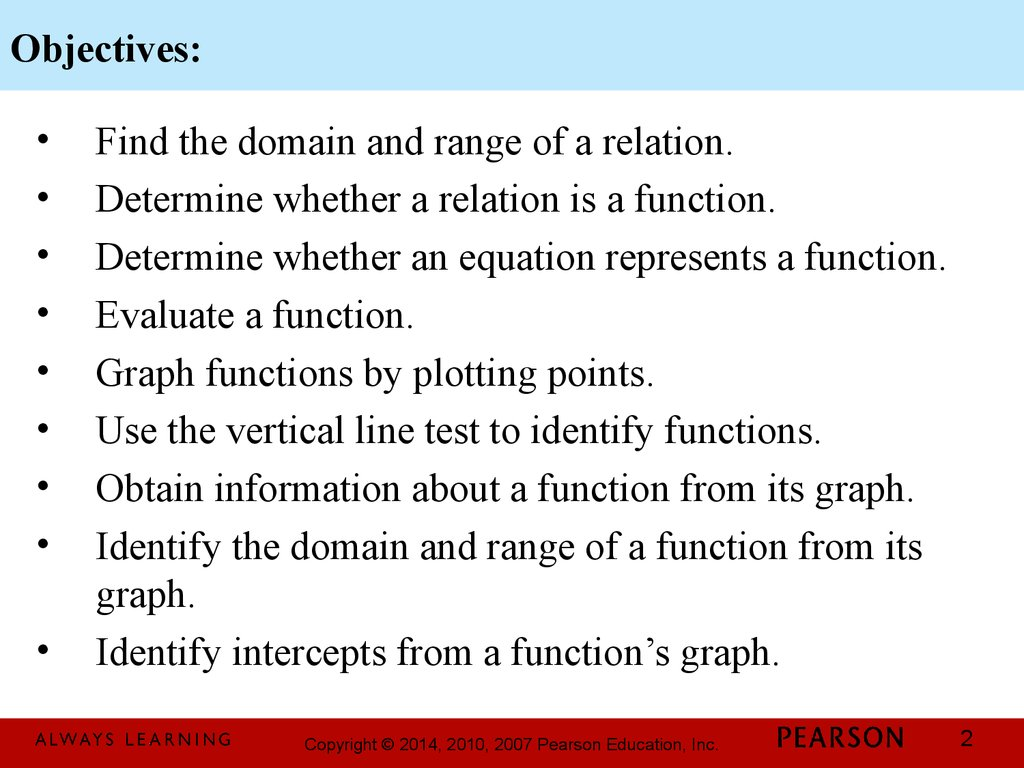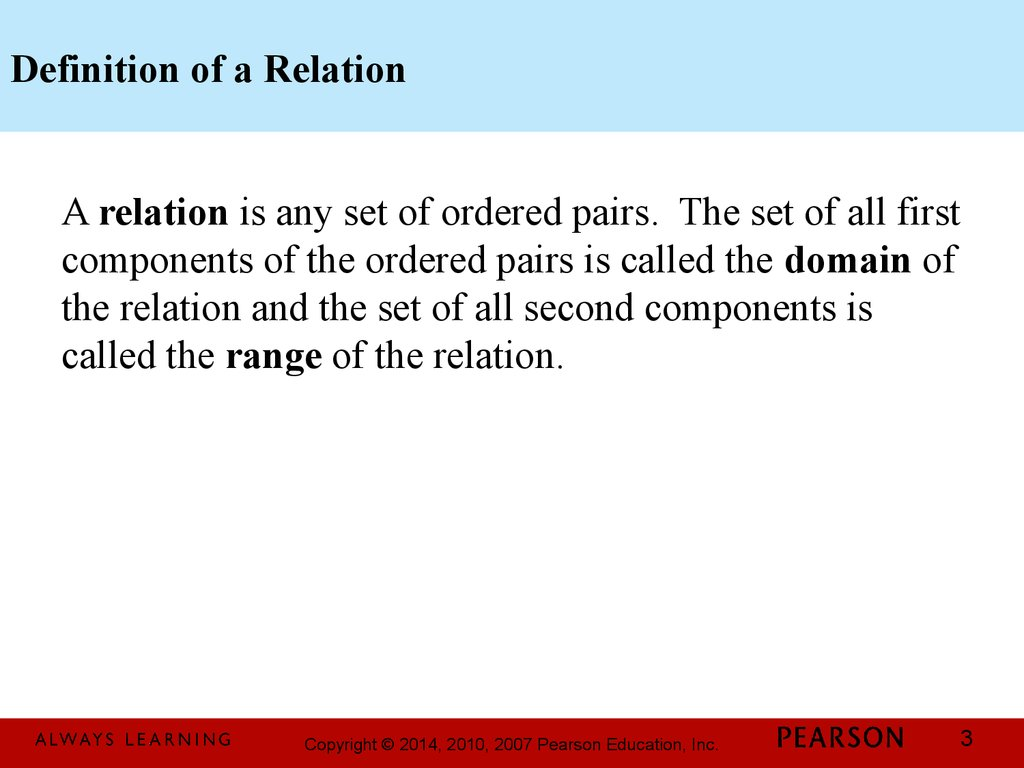# Basics of functions and their graphs

## 1.

Chapter 2
Functions and
Graphs
2.1 Basics of Functions
and Their Graphs
1

## 2. Objectives:

Find the domain and range of a relation.
Determine whether a relation is a function.
Determine whether an equation represents a function.
Evaluate a function.
Graph functions by plotting points.
Use the vertical line test to identify functions.
Obtain information about a function from its graph.
Identify the domain and range of a function from its
graph.
Identify intercepts from a function’s graph.
2

## 3. Definition of a Relation

A relation is any set of ordered pairs. The set of all first
components of the ordered pairs is called the domain of
the relation and the set of all second components is
called the range of the relation.
3

## 4. Example: Finding the Domain and Range of a Relation

Find the domain and range of the relation:
{(0, 9.1), (10, 6.7), (20, 10.7), (30, 13.2), (40, 21.2)}
domain: {0, 10, 20, 30, 40}
range: {9.1, 6.7, 10.7, 13.2, 21.2}
4

## 5. Definition of a Function

A function is a correspondence from a first set, called
the domain, to a second set, called the range, such that
each element in the domain corresponds to exactly one
element in the range.
5

## 6. Example: Determining Whether a Relation is a Function

Determine whether the relation is a function:
{(1, 2), (3, 4), (6, 5), (8, 5)}
No two ordered pairs in the given relation have the
same first component and different second components.
Thus, the relation is a function.
6

## 7. Functions as Equations

If an equation is solved for y and more than one value of
y can be obtained for a given x, then the equation does
not define y as a function of x.
7

## 8. Example: Determining Whether an Equation Represents a Function

Determine whether the equation defines y as a function
of x.
x2 + y 2 = 1
y2 = 1 - x2
y = ± 1 - x2
The ± shows that for certain values of x, there are two
values of y. For this reason, the equation does not
define y as a function of x.
8

## 9. Function Notation

The special notation f(x), read “f of x” or “f at x”,
represents the value of the function at the number x.
9

## 10. Example: Evaluating a Function

If f ( x) = x - 2 x + 7, evaluate f (-5).
2
f ( x) = x 2 - 2 x + 7,
f (-5) = (-5) 2 - 2( -5) + 7 = 25 + 10 + 7 = 42
Thus, f (-5) = 42.
10

## 11. Graphs of Functions

The graph of a function is the graph of its ordered
pairs.
11

## 12. Example: Graphing Functions

Graph the functions f(x) = 2x and g(x) = 2x – 3 in the
same rectangular coordinate system. Select integers for
x, starting with –2 and ending with 2.
12

## 13. Example: Graphing Functions (continued)

We set up a partial table of coordinates for each
function. We then plot the points and connect them.
f ( x) = 2 x
g ( x) = 2 x - 3
x
y = f (x)
x
y = f (x)
–2
–4
–2
–7
–1
–2
–1
–5
0
0
0
–3
1
2
1
–1
2
4
2
1
13

## 14. The Vertical Line Test for Functions

If any vertical line intersects a graph in more than one
point, the graph does not define y as a function of x.
14

## 15. Example: Using the Vertical Line Test

Use the vertical line test to identify graphs in which y is
a function of x.
not a function
function
15

## 16. Example: Analyzing the Graph of a Function

Use the graph to find f(5)
f(5)=400
For what value of x
is f(x) = 100?
f(9) = 125, so x = 9.
16

## 17. Identifying Domain and Range from a Function’s Graph

To find the domain of a function from it’s graph,
look for all the inputs on the x-axis that correspond to
points on the graph.
To find the range of a function from it’s graph, look
for all the outputs on the y-axis that correspond to points
on the graph.
17

## 18. Example: Identifying the Domain and Range of a Function from Its Graph

Use the graph of the function to identify its domain and
its range.
Domain { x -2 £ x £ 1}
[-2,1]
Range
{ y 0 £ y £ 3}
[0,3]
18

## 19. Example: Identifying the Domain and Range of a Function from Its Graph

Use the graph of the function to identify its domain and
its range.
Domain
{ x -2 < x £ 1}
(-2,1]
Range
{ y -1 £ y < 2}
[-1,2)
19

## 20. Identifying Intercepts from a Function’s Graph

To find the x-intercepts, look for the points at which
the graph crosses the x-axis.
To find the y-intercept, look for the point at which the
graph crosses the y-axis.
A function can have more than one x-intercept but at
most one y-intercept.
20

## 21. Example: Identifying Intercepts from a Function’s Graph

Identify the x- and y-intercepts for the graph of f(x).
·
·
·
·
The x-intercepts are
(–3, 0)
(–1, 0)
and (2, 0)
The y-intercept is (0, –6)